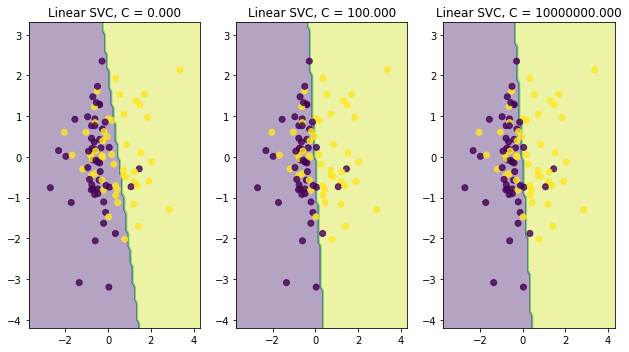I would like you to go through Intuition Behind SVM before exploring about Regularization.

SVM has an objective To find the optimal linearly speparating hyperplane which maximizes the margin. But, we know that Hard-Margin SVM can work well when data is completely lineary separable (without any noise or outliers). What if our data is not perfectly separable? We have two options for non-separable data:

 a. Using Hard-Margin SVM with feature transformations
b. Using Soft-Margin SVM

If you want a good generalization on your result, we should tolerate some errors. If we force our model to be perfect, it will be just an attempt to overfit the data!.

Let's talk about Soft-Margin SVM and it helps us to understand Regularization. If the training data is not linearly separable, we allow our hyperplane to make few mistakes on outliers or say noisy data. Mistakes means those outliers/noise data can be inside the margin or on the wrong side of the margin.

But, we will have a mechanism to pay a cost for each of those misclassified example. That cost will depend on how far the data point is from the margin. This cost is represented by slack variables ($ξ_i$)

objective function : $\frac{1}{2} ||w||^2 + C \sum_{i=1}^{n} ξ_i$

In the above equation, the parameter C defines the strength of regularization. We can discuss three different cases based on values of C:

1. Small C :

• $||w||^2$ dominates the objective function.
• Forces towards finding a large margin hyperplane.
• Allows violations/misclassified training examples.
• i.e Ignoring outliers/ noises in the data.
2. Large C :

• $C \sum_{i=1}^{n} ξ_i$ dominates the expression.
• Forces to fit all the training data, which leads to hyperplane with smaller margin.
• Since all data points are taken to be important for classifier, it overfits the data.
3. C = $\inf$

• It enforces all constraints, and we will get Hard-Margin SVM.

Let's try to see the output with some randomly generated data using sklearn

In :
import numpy as np
import matplotlib.pyplot as plt

from sklearn.datasets import make_classification
from sklearn.svm import SVC

In :
# HELP FROM : https://scikit-learn.org/stable/auto_examples/ensemble/plot_voting_decision_regions.html

def plot_decision_boundary(model, X, y, title, subplot):

# MESH STEP SIZE
h = .02
x_min, x_max = X[:, 0].min() - 1, X[:, 0].max() + 1
y_min, y_max = X[:, 1].min() - 1, X[:, 1].max() + 1
xx, yy = np.meshgrid(np.arange(x_min, x_max, h), np.arange(y_min, y_max, h))

Z = model.predict(np.c_[xx.ravel(), yy.ravel()])

x_min, x_max = X[:, 0].min() - 1, X[:, 0].max() + 1
y_min, y_max = X[:, 1].min() - 1, X[:, 1].max() + 1
xx, yy = np.meshgrid(np.arange(x_min, x_max, 0.1),
np.arange(y_min, y_max, 0.1))

Z = model.predict(np.c_[xx.ravel(), yy.ravel()]).reshape(xx.shape)

subplot.contourf(xx, yy, Z, alpha=0.4)
subplot.scatter(X[:, 0], X[:, 1], c=y, alpha=0.8)
subplot.set_title(title)

X, y = make_classification(n_samples = 100, n_features=2,
n_redundant=0, n_informative=2,
class_sep = 0.5, random_state=0)

fig, subplots = plt.subplots(1, 3, figsize=(9, 5))

clf1 = SVC(C=0.0001, kernel='linear').fit(X, y)
title = 'Linear SVC, C = {:.3f}'.format(0.0001)
plot_decision_boundary(clf1, X, y,title, subplots)

clf2 = SVC(C=10.0, kernel='linear').fit(X, y)
title = 'Linear SVC, C = {:.3f}'.format(100.0)
plot_decision_boundary(clf2, X, y,title, subplots)

clf3 = SVC(C=100000.0, kernel='linear').fit(X, y)
title = 'Linear SVC, C = {:.3f}'.format(10000000.0)
plot_decision_boundary(clf3, X, y,title, subplots)

plt.tight_layout()Main points to remember:

   1. Hard-Margin SVM is not robust to outliers or noisy data points.
2. In order to solve this, we use Soft-Margin SVM classifier, where we allow some violations and we penalize the   sum of violations in the objective functions.
3. 'C' is the regularization parameter which maintains the tradeoff between the size of the margin and violations of the margin.


(i.e forces towards smaller $||w||^2$ and smaller $\sum_{i=1}^{n} ξ_i$ )

In [ ]: